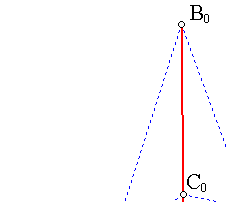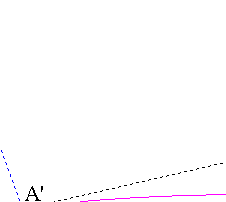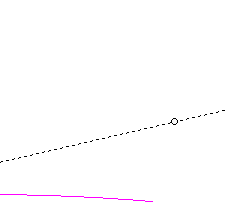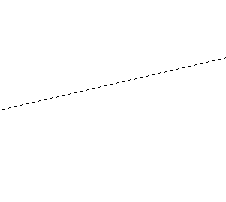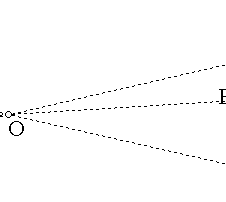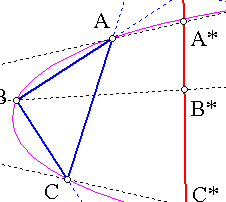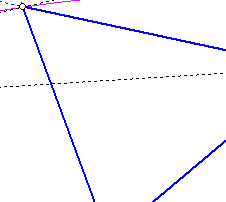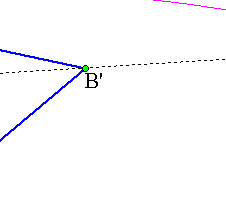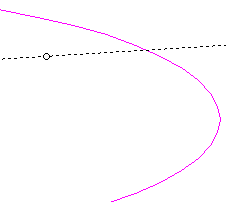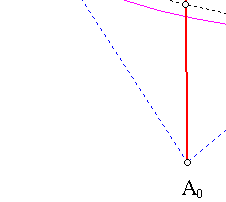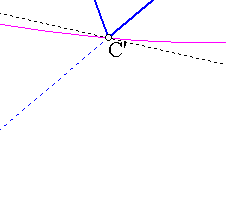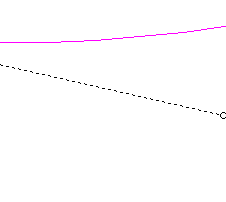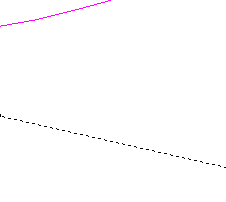##1. Harmonic perspectivities

Harmonic perspecivities are projectivities which posses a line of fixed points (e), an isolated fixed point O not lying on (e) and the property that for every point X other than O its image Y satisfies                            (X, Y, O, HX) = -1,
where HX is the intersection point of OX with (e). Later condition meaning that (X,Y) are harmonic conjugate with respect to (O, HX), and of course all four points lying on line OX.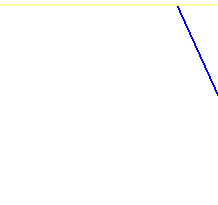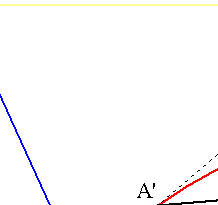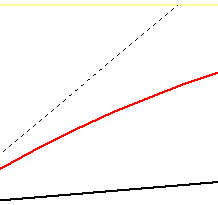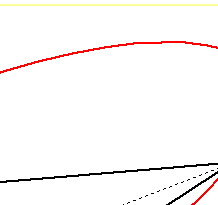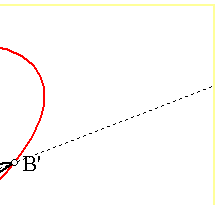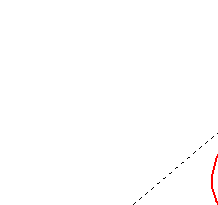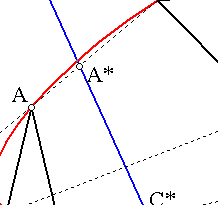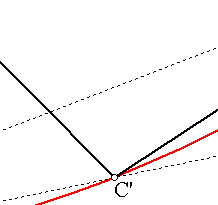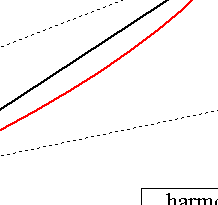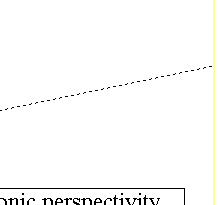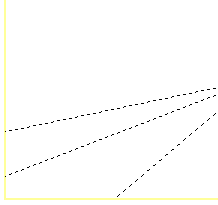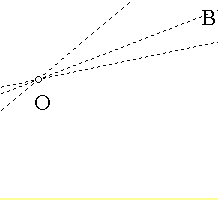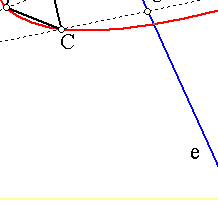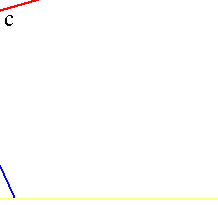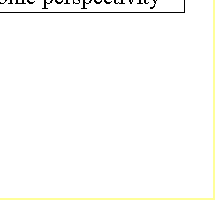##2. Harmonic perspective triangles

Given a perspectivity f(O,e) (with center O and axis e), taking a triangle ABC and its image A'B'C' = f(ABC) we obtain two perspective triangles with perspectivity axis e.
Two such triangles are called harmonic perspective.
Every conic passing through the vertices A,A',C',C has the pair (O,e) as a pair of pol-polar. Thus, if B lies on such a conic, then necessarily also B' will lie on c.
This implies that:
Through the vertices of every pair of harmonic perspective triangles passes a conic, which is invariant under the perspectivity defined by the two triangles.

##3. Perspective triangles having the vertices on a conic

The inverse of the previous proposition is also true. If two triangles are perspective and their vertices lie on a conic, then the perspectivity they define is harmonic and the pair (O,e) of center and axis of perspectivity are respectively (pol, polar) of the conic.

By  the general properties of perspectivities (see Perspectivity.html ), the two perspective triangles define a perspectivity. By the same general properties A'C and AC' intersect on e and corresponding property holds also for the pairs of line (BC', B'C) and (A'B, AB'). It follows that e is the polar of O, thereby proving the claim.

##4. General perspective triangles

In general, two perspective triangles ABC and A'B'C', having lines AA', BB', CC' meeting at the perpsectivity center O, define a perspectivity with homology ratio k different from -1. This constant k is the common cross ratio:
k = (A,A',O,A*) = (B,B',O,B*) = (C,C',O,C*),
defined along lines OA, OB, OC by their corresponding intersections A*, B*, C* with the perspectivity axis.
There is a conic passing through all six vertices of the triangles precisely when this k = -1.
A special case of perspective triangles is examined in the file Harmonic_Perspective_Triangles.html .